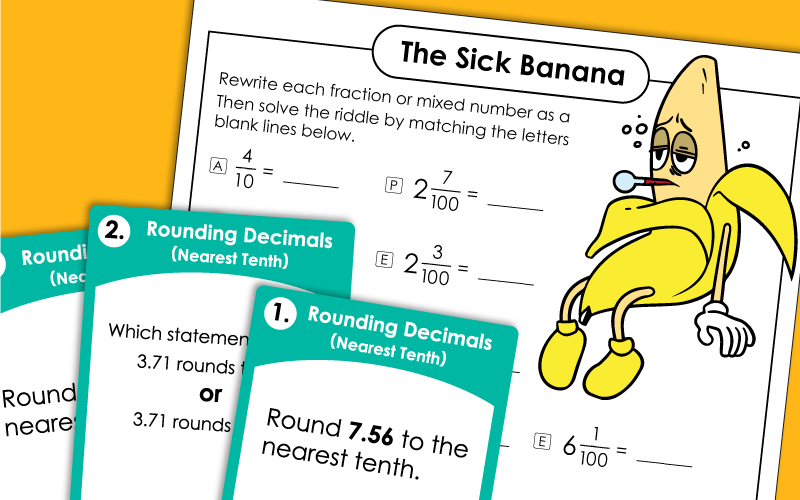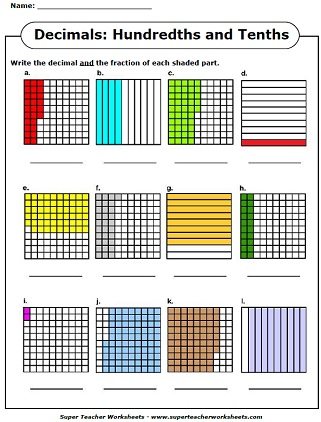# Decimal Worksheets

These worksheets can help your students review decimals number concepts.  Worksheets include place value, naming decimals to the nearest tenth and hundredth place, adding decimals, subtracting decimals, multiplying, dividing, and rounding decimals.## Tenths

Tell what part of each graphic is shaded.  Decimals to the nearest tenth.  (example: 0.6)
Cut out the decimal cards and lay them on the table in order from least to greatest.
Write each mixed number or fraction as a decimal and vice-versa.
Convert the written words to decimal numbers and fractions or mixed numbers.
Fill in the fraction (mixed number), the decimal number, or the decimal word name.
Write the value of the underlined digit for each decimal number.
Practice writing and reading numbers on this place value chart.  Includes thousands, hundreds, tens, ones, and tenths.
Use this set of task cards to practice rounding decimals to the nearest tenth.

## Hundredths

Write the decimal and fraction that is shown by each picture.
Write the fraction and decimal number that tells what part of the boxes is shaded.
Place the number cards in order from smallest to largest.  Includes five sets on number cards with whole numbers and hundredths decimals.
Switch the written decimal words into decimal numbers.
Fill in the missing decimal number, the fraction (mixed number) or the word name.
Round decimals to the nearest tenth and to the nearest whole number.
Determine the value of the underlined digit for each decimal.
Write decimal numbers on this chart with place value columns. Place value columns range from hundred thousands to hundredths.

## Thousandths

Determine the value of the underlined digit for each decimal.
Students can write large decimals on this place value chart.  Place value columns range from millions to thousandths.
Rewrite the decimals shown in order, from least to greatest. Includes decimals up to thousandths.
Cut out the cards and order them from least to greatest. Numbers are decimals with a thousandths place.
Why did the clock get in trouble at school? To find out, rewrite each mixed number as a decimal. Then use the answers to decode the solution to the riddle.
Read each decimal word name and rewrite the decimal in standard form.
Just like the above Reading Numbers Wheel, but includes thousandths also. Students cut out the two wheel halves, fasten them together, and spin, reading the displayed number aloud. Includes 6 score sheets for teachers.

## Tenths & Hundredths Mixed

Look at the picture and write the decimal and fraction.  Includes tenths and hundredths.
Complete the missing numbers on this hundredths and tenths table.
Thirty task cards on identifying decimals names and numbers, write numbers in decimal notation. Includes tenths and hundredths.
Students cut out the two wheel halves, fasten them together, and spin, reading the displayed number aloud. Includes 6 score sheets for teachers.
Cut the decimal cards apart and arrange the numbers in order from least to greatest.
Convert each mixed number into a decimal. Then use the answers to find the answer to the funny riddle. Why did the banana see his doctor? Because he wasn't peeling very well.
Cut out the fraction cards. Glue each next to its corresponding decimal.
Students must tell which numbers are decimals, which are fractions, and which are mixed numbers.
Match the decimal or number on the left with the word name on the right.
Use the symbols <, >, and = to compare the decimal numbers.  (tenths and hundredths)
Use greater than, less than, and equal to symbols to show which decimal is bigger.  Decimals are in tenths and hundredths.  (example: 1.01 < 1.11)
This worksheet has a section of comparing decimals, a section of ordering decimals, and a word problem.
Part 1:  Write each number as a decimal.  Part 2:  Write the word name for each decimal.  Part 3:  Add or subtract the decimal numbers (hundredths and tenths)

## Also on S.T.W...

Compare and Order Decimals

This section of our website has a set of worksheets for teaching students to order and compare decimal numbers.

Add and subtract decimal numbers with these printable worksheets.

Multiplying Decimals

Learn to multiply pairs of numbers with decimal factors.

Dividing Decimals

Practice long division math problems with decimals in the dividend and/or divisor.

Fraction Worksheets

This page has basic fractions, fractions of a set, comparing and ordering fractions, and more.

Full Math Worksheet Index

View an index of all math worksheets available on Super Teacher Worksheets.

## Sample Pictures ofDecimal ActivitiesMy Account
Site Information